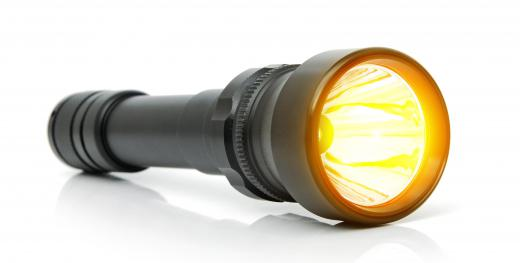Science
Fact Checked

# What is a Lumen?

Brendan McGuigan
Brendan McGuigan

A lumen is a unit of standard measurement used to describe how much light is contained in a certain area. It is part of a group of standard measurements known as the photometry group, which measure different aspects of light. This group also includes such units as the candela, which measures luminance, and the lux, which measures illuminance.

Strictly speaking, a lumen is defined as 1 candela multiplied by 1 steradian, which can be expressed as . A related unit of measurement — although not part of the standard units — is the foot-candle, which is often used in photography and film. To really understand this, it is important to understand these units: the candela, the foot-candle, the steradian, and the lux.Lumen is the total amount of visible light emitted from a source.

There is an important distinction to be made between measures of radiance and measures of illuminance. When measuring radiance, someone is basically looking at how much energy a light source is releasing directly. He is not interested in what happens to that energy as it leaves the source, just in how much is at the source itself. When measuring illuminance, by contrast, a person is looking at how much of that energy makes it to a given object.

The foot-candle, also spelled footcandle, is the non-standard measure of illuminance, basically defined as how much light would strike the inner surface of a sphere 1 foot (30.48 cm) in radius if it were lit by a single candle in the center of that sphere. The lux is the standard measure of illuminance, and can be defined simply as how much light would strike a similar sphere of 3.28 feet (1 meter) in radius. The two can be converted simply, with 10.76 lux to 1 foot-candle.

Both the foot-candle and the lux make use of a measure of luminous intensity, called the candela. The candela was originally called the candlepower and simply referred to the amount of luminous energy emitted by a known type of candle. Later, when it was standardized, a definition was chosen that would approximate the older definition, so that existing equations could remain the same. The modern definition of the candela has to do with the radiation emitted by 1/60 of a single square centimeter of platinum when it is at its melting point.

A steradian is a standard unit of measurement used to define a solid angle. The technical definition is "the solid angle subtended at the center of a sphere of radius r by a portion of the surface of the sphere having an area of r2." In this definition, subtended has to do with the relationship between the length of the arc and the resulting angle. The steradian is unitless and is represented by the abbreviation sr. Mathematically, using the meter as a unit of measurement, we can then define a steradian as 1(sr) = m2 x m-1.

So, taking all of this together, it is easier to interpret the original definition of a lumen as 1(lm) = 1(cd) x 1(sr). One lumen, in this case, is a measure of the amount of luminous flux emitted into an area by 1/60 of a single square centimeter of platinum at its melting point into a certain angle. This measurement is often used along with wattage to determine the luminous efficiency of a light-emitting body.

For example, a normal 100 watt light-bulb has a luminous efficiency of about 17.5, emitting 1750 lumens of light. A 13 watt fluorescent bulb, by contrast, has an efficiency of about 56, emitting around 730 lumens of light. The sun, in contrast, has an efficiency of around 93.

## You might also Likeanon351898

What we in the west will have to do is just recalibrate our life's experiences with brightness to lumens instead of watts. Anon2634 asks a complex question with little specificity. But let me just take a stab in the dark for an answer.

If I am sitting in a pew with my bible open in the dark, what light bulb would I need to choose to be directly 3 meters above my head to achieve enough light to read by? My guess would be Sylvania ULTRA RT 6 LED10W Downlight 700 lumens.

anon26343

What is the best no. of lumens in a big church or a cathedral?

anon16230

Here's a simpler explanation: 1 lux is the light level you get when you hold a lit candle one meter from the subject in a dark room. If you hold the candle just a foot away the illumination is brighter, of course, and we call that lighting level one foot-candle, or about 10.76 lux. (In practice 10 lux = 1 footcandle is close enough.)

To light a surface of one square meter evenly at a 1 lux requires one lumen of total light. Since the area of a sphere one meter in radius is 4pi square meters, the total light output of a candle is 4pi (about 12.6) lumens. So a candle is a little dimmer than a one watt light bulb.

A candela is a measure of light intensity per unit angle, specifically one lumen per steradian, but hardly anybody actually has to know that.... - D Gary Grady